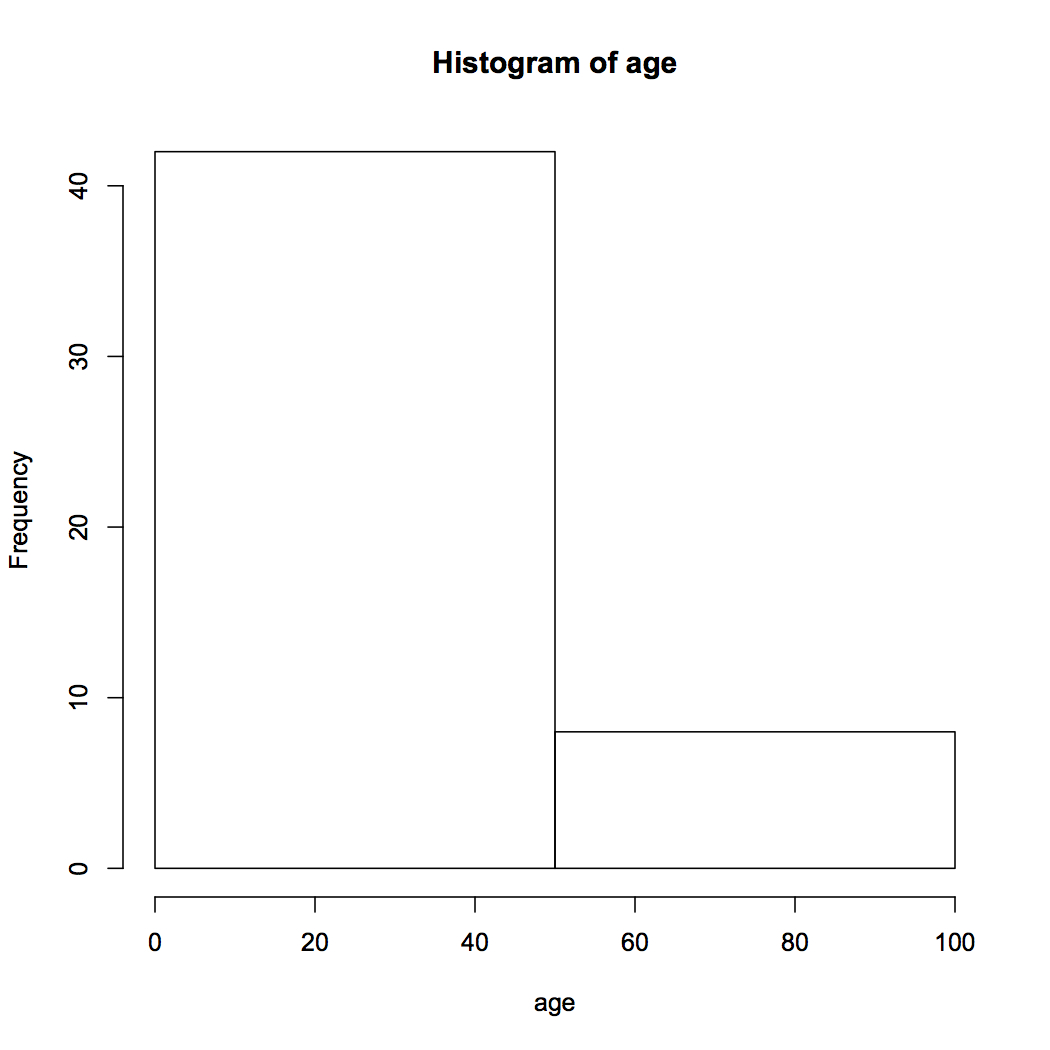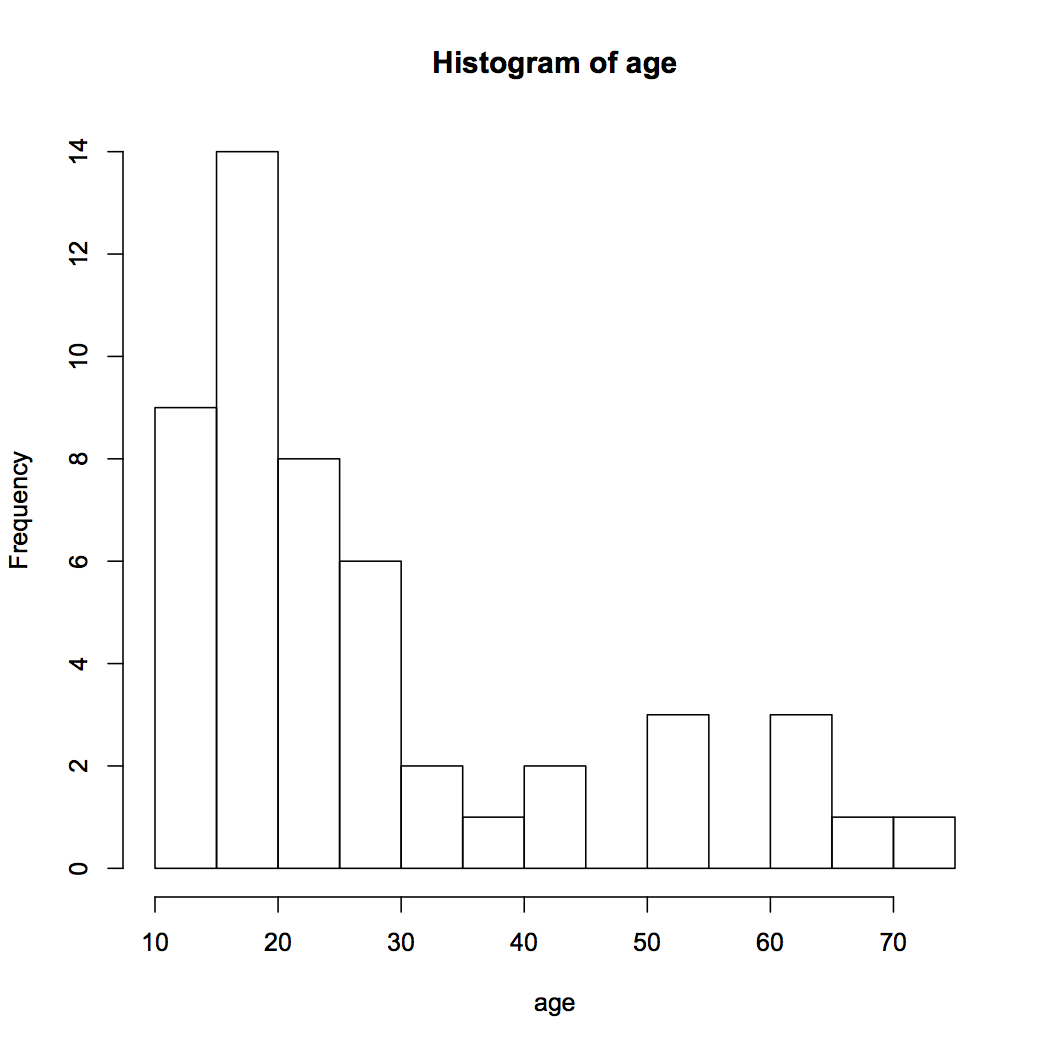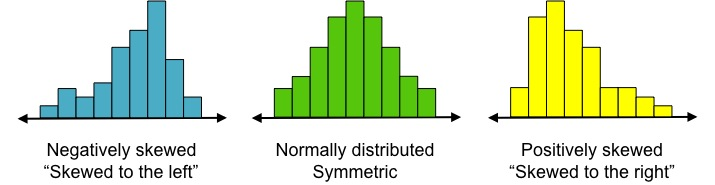PURCHASE A DIGITAL COPY PURCHASE A HARD COPY Lesson 1 Introduction to Statistical Research Methods Lesson 2 Visualizing Data Lesson 3 Central Tendency Lesson 4 Variability Lesson 5 Standardizing Lesson 6 Normal Distribution Lesson 7 Sampling Distributions Lesson 8 Estimation Lesson 9 Hypothesis Testing Lesson 10 t-Tests for Dependent Samples Lesson 11 t-Tests for Independent Samples Lesson 12 Intro to One-Way ANOVA Lesson 13 One-Way ANOVA: Test significance of differences Lesson 14 Correlation Lesson 15 Linear Regression Lesson 16 Chi-Squared Tests Afterward Index

When we have a bunch of data, we must organize and visualize it in order to make sense of it. Hans Rosling, a Swedish doctor, mathematician, and professor, has a great video showing one of the earliest methods used to visualize data.

A standard way to visualize categorial data is by finding the frequency of different occurrences. For example, let’s say we have a random sample of 50 statistics students and the country in which they live.

In this spreadsheet, each row represents one student (except Row 1, which is the header). For example, the student represented by Row 2 is from the United States.

If we want to know the most common country in which statistics students live, we should make a frequency table by counting the number of occurrences of each country, e.g.

 Country Frequency China 12 US 10 India 8 Japan 8 Germany 3 Mexico 3 Other 6

We can do this manually [quiz], or use a statistical program. Let’s see how it’s done using R.

After finding the frequencies, we can visualize this data with a bar chart.Now we can see in a glance that most students are from China. That’s the beauty of data visualization — we can more easily and quickly see patterns in the data.

Sometimes we want to know the distribution of percentages or proportions to quickly see the composition of the sample or population. For example, we want to know what percent of students in this sample are from China. While frequencies are absolute numbers, percentages and proportions are relative numbers. These are called relative frequencies, which we find by dividing each frequency by the total number.

 Relative Frequency: The percentage or proportion of the total number with that characteristic, found by dividing the absolute frequency by the total number in the population. [video] [quiz]

Let’s add relative frequency columns to the table we’ve started.

 Country Frequency (absolute) Percentage (relative) Proportion (relative) China 12 12/50 = 24% 12/50 = 0.24 US 10 10/50 = 20% 10/50 = 0.20 India 8 8/50 = 16% 8/50 = 0.16 Japan 8 8/50 = 16% 8/50 = 0.16 Germany 3 3/50 = 6% 3/50 = 0.06 Mexico 3 3/50 = 6% 3/50 = 0.06 Other 6 6/50 = 12% 6/50 = 0.12 Total 50 50/50 = 100% 50/50 = 1.00

Note that percentages range from 0% to 100% and should add to 100%. Proportions range from 0 to 1 and should add to 1.

Country is a categorical variable, as opposed to a numerical variable. What if our variable of interest was numerical, as in the student_ages dataset, and we wanted to create a frequency table? Now we would have to choose an interval length (also called “bin size”) for our x-axis values. The y-axis would be the number of subjects that fall within that interval. In this case, it would be the number of students whose ages are within each interval. In the lesson, we gave the example of intervals of length 20 and created a frequency table. [video] [quiz]

We can then visualize frequencies with a histogram. A histogram is very similar to a bar chart; however, the x-axis is numerical and continuous, and intervals are adjacent. Let’s learn how to create histograms in R.

 R Tutorial: Creating histograms for numerical data Again, let’s first input the dataset into R. Open the student_ages dataset. Go to File > Download as > Comma Separated Values Move the file from your Downloads folder to your working directory and rename it student_ages.csv. Import the data into R: student_ages = read.csv(file = “student_ages.csv”, head = TRUE, sep = “,”) Next, we need R to recognize “age” as the name of the variable using the attach() function. Then we can create a histogram of “age” with the hist() function. attach(student_ages) hist(age) R automatically created intervals of length 10. Now we can easily see that most students are between ages 10 and 20.We can make these intervals smaller or larger by specifying the number of “breaks” in the code. For example, hist(age, breaks = 2) will create two intervals of size 50:while  hist(age, breaks = 20) created a lot more intervals:Check out this app to play around with different bin sizes. (You may need to use Safari or Firefox.)

You should see that as we decrease the bin size, we can be much more precise in our frequency calculations. For example, with the small bin sizes in the histogram above, we can see that most students are between age 16 and 20, whereas with just two breaks, we can only conclude that most students are less than 50 years old (not a very helpful conclusion).

Another important thing about histograms is their shape. Our sample of Udacity students’ ages was positively skewed (also called “skewed to the right”) because the majority of values occurred on the left of the distribution and the long “tail” of the distribution is on the right.Distributions that are symmetric are called “normal” (middle histogram). Below are some real-life examples of data of each shape:

 Negatively skewed Human life expectancy (most people live to be at least 50) Normally distributed Height Positively skewed Income